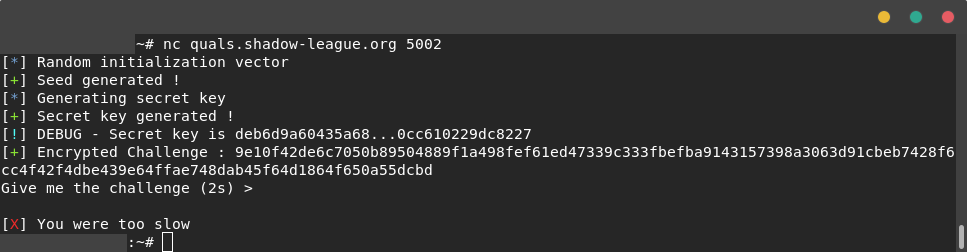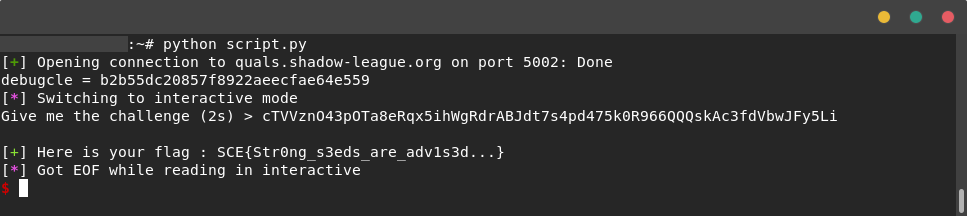# Challenge Accepted !

Quals Sogeti Cyber E-scape 2019 - Prog (493 pts).

### Challenge details

Event Challenge Category Points Solves
Quals Sogeti Cyber E-scape 2019 Challenge Accepted ! Programmation 493 51

### Description (original)

Serez-vous capable de battre la machine ? Celle-ci est programmée pour vous envoyer un challenge chiffré. Votre tâche est de déchiffrer ce challenge et le renvoyer à la machine en moins de 2 secondes.

Une copie du programme vous est fournie.

`nc quals.shadow-league.org 5002`

### Description [EN]

Will you be able to beat the machine? This one is programmed to send you an encrypted challenge. Your task is to decipher this challenge and send it back to the machine in less than 2 seconds.

A copy of the program is provided.

`nc quals.shadow-league.org 5002`

### TL;DR

This was a hash bruteforce due to random.seed(N), where N is in a given range.

### Methology

First thing I did was looking at the python source code:

``````import random
import hashlib
import string
import time
from Crypto.Cipher import AES

CHALLENGE = ''.join(random.choice(string.ascii_lowercase + string.ascii_uppercase + string.digits) for _ in range(64))

FLAG = "SCE{DEMO PROGRAM - NOTHING HERE}"

class bcolors:
OKBLUE = '\033[94m'
OKGREEN = '\033[92m'
WARNING = '\033[93m'
FAIL = '\033[91m'
ENDC = '\033[0m'

def pprint(message, level="INFO"):
if level == "INFO":
print("[" + bcolors.OKBLUE + "*" + bcolors.ENDC + "] " + message)
elif level == "WARNING":
print("[" + bcolors.WARNING + "!" + bcolors.ENDC + "] " + message)
elif level == "FAIL":
print("[" + bcolors.FAIL + "X" + bcolors.ENDC + "] " + message)
elif level == "SUCCESS":
print("[" + bcolors.OKGREEN + "+" + bcolors.ENDC + "] " + message)

pprint("Random initialization vector", "INFO")
random.seed(random.randint(1,10000))
pprint("Seed generated !", "SUCCESS")

key = 0xffffffff

pprint("Generating secret key", "INFO")
for i in range(10):
key ^= random.randint(0x00000000, 0xffffffff)
pprint("Secret key generated !", "SUCCESS")

secret = hashlib.sha256(str(key))

# @TODO REMOVE THIS IN PRODUCTION !
pprint("DEBUG - Secret key is %s...%s" % (secret.hexdigest()[:15], secret.hexdigest()[-15:]), "WARNING")

encryption_suite = AES.new(secret.digest(), AES.MODE_CBC, 'LmQHJ6G6QnE5LxbV')
cipher_text = encryption_suite.encrypt(CHALLENGE)

pprint("Encrypted Challenge : " + cipher_text.encode("hex"), "SUCCESS")

current_time = time.time()
USER_CHALLENGE = raw_input("Give me the challenge (2s) > ")

if time.time() - current_time > 2:
pprint("You were too slow", "FAIL")
else:
if USER_CHALLENGE == CHALLENGE:
pprint("Here is your flag : " + FLAG, "SUCCESS")
else:
pprint("Incorrect challenge", "FAIL")
``````

Here is a screen of the TCP connection on the challenge:We can see that the code is creating a key named `"key"` with multiple random and xor call. Then, the key is hashed and renamed `"secret"` with sha256 and partially displayed to the user (15 first chars and 15 last chars). Finally this key is used to encrypt a secret challenge with AES and the IV `encryption_suite`. To solve the challenge, the user have 2 seconds to give the decrypted challenge, knowing the algorithm and part of the key.

#### Identify potential vulnerabilities

At my first look on the code I thought about a first solution: As long as the `secret` is the sha256 of an integer between 0x00000000 and 0xffffffff, we just need to compute all the hashes and pick the right one when we connect to the challenge. This solution would have been possible but quite long.

At my second look on the code, I’ve notice the ‘seed’ keyword:

``````random.seed(random.randint(1,10000))
``````

When the seed is known, random output is predictible and replayable. Here, we know that the seed is an integer between 1 and 10000. So we can compute the 10000 secret (1 per seed) then pick the right one when we connect to the challenge !

Here is the generation of a dictionnary with the 10000 secret and their partial key as dictionnary key.

``````sdico = {}
for i in range(1,10001):  # Generate the 10000 key
random.seed(i)
key = 0xffffffff
for j in range(10):
key ^= random.randint(0x00000000, 0xffffffff)
secret = hashlib.sha256(str(key).encode("utf-8"))
s = secret.hexdigest()[:15]+secret.hexdigest()[-15:]  # get displayed part of the key
sdico[s] = secret  # add secret in dictionnary with displayed part of the key as dictionnary key
``````

Now we need to link the code with the challenge. I used pwnlib instead of socks. I also realised that the code was running in Python2 because Python2 and Python3 does’nt share the same random PRNG.

Final code:

``````# -*- coding:utf-8 -*-

from pwn import *
import random
import hashlib
import string
import time
from Crypto.Cipher import AES

sdico = {}
for i in range(1,10001):  # Generate the 10000 key
random.seed(i)
key = 0xffffffff
for j in range(10):
key ^= random.randint(0x00000000, 0xffffffff)
secret = hashlib.sha256(str(key).encode("utf-8"))
s = secret.hexdigest()[:15]+secret.hexdigest()[-15:]  # get displayed part of the key
sdico[s] = secret  # add secret in dictionnary with displayed part of the key as dictionnary key

conn = remote("quals.shadow-league.org",5002)  # Connection au serveur distant

conn.recvuntil("key is")
debugcle = conn.recvuntil("\r\n").strip().replace(".","")
print("debugcle = "+str(debugcle))
cle = sdico[debugcle].digest()

conn.recvuntil("Challenge : ")
CHALLENGE = conn.recvuntil("\r\n").strip()
CHALLENGE = CHALLENGE.decode("hex")  # Recup "Encrypted Challenge"

encryption_suite = AES.new(cle, AES.MODE_CBC, "LmQHJ6G6QnE5LxbV")
cipher_text_decode = encryption_suite.decrypt(CHALLENGE)

conn.send(cipher_text_decode+"\r\n")
conn.interactive()
``````#### Flag

`SCE{Str0ng_s3eds_are_adv1s3d...}`

Zeecka